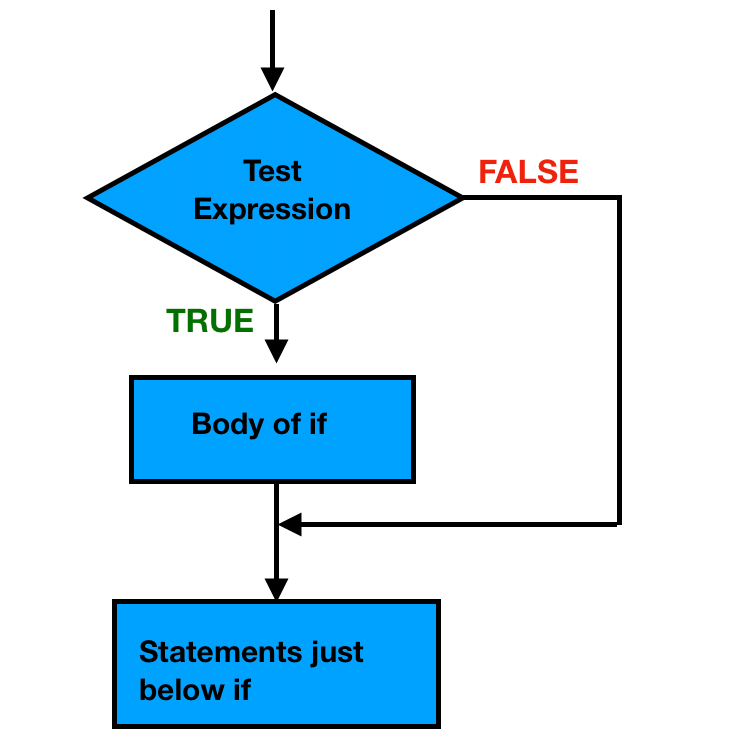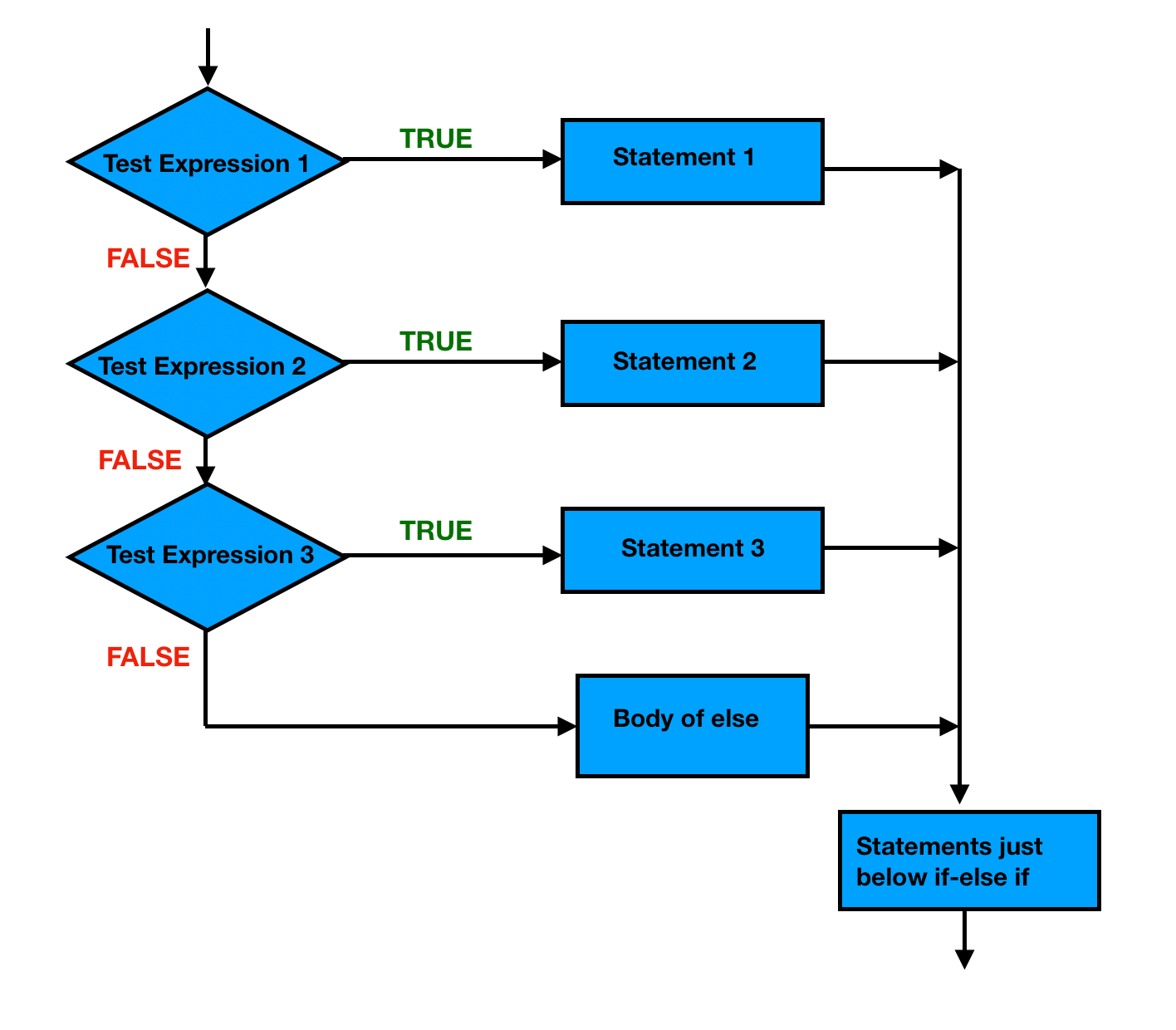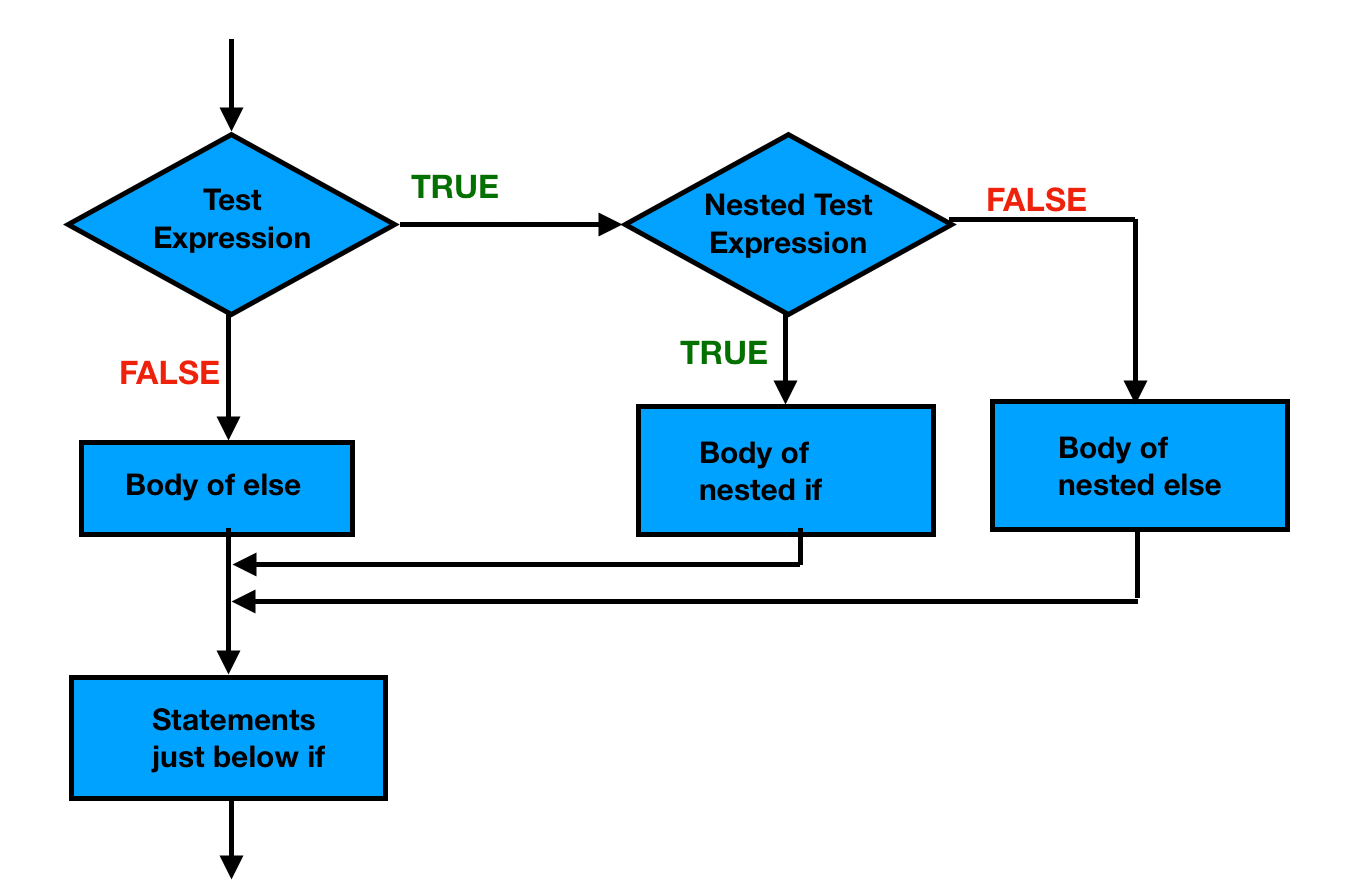# Java if…else

In this article, you will learn to use selection statements if, if-else, if-else-if to control the flow of execution of a program based on certain conditions.

Selection statements also known as conditional statements allows a program to test several conditions, and execute instructions based on which condition is true.

#### The conditional statements:

• if statement
• if-else statement
• Nested if

### if statement

The if statement checks the given condition. If the condition evaluates to be true then the block of code/statements will execute otherwise not.

#### Syntax

The syntax of if statement:

```if (condition) {
// block of code to be executed if the condition is true
}```

The condition is a boolean expression (returns either true or false).

If the condition is evaluated to true, statements inside the body of if (statements inside curly braces) gets executed.

If the expression is evaluated to false, statements inside the body of if are skipped from execution.

#### Flowchart:#### Example

```int x = 20;
int y = 18;
if (x > y) {
System.out.println("x is greater than y");
}
System.out.println("Outside if statement");```

#### Output

```x is greater than y
Outside if statement```

#### Example explained

In the above example, when x=20 and y=18:

• condition 20>18 is checked, yields true and x is greater than y” gets printed.

when x=18 and y=20:

• condition 18>20 is checked, yields false.
• the Java compiler skips the execution of the body of if statement and  “Outside if statement” gets printed.

### if-else statement

The if statement executes a certain section of code if the test expression is evaluated to true. The if statement may have an optional else block. Statements inside the body of else statement are executed if the test expression is evaluated to false.

#### Syntax

The syntax of if-else statement:

```if (condition) {
// block of code to be executed if the condition is true
} else {
// block of code to be executed if the condition is false
}```

If the condition is evaluated to true, statements inside the body of if are executed.

If the condition is evaluated to false, statements inside the body of else are executed.

#### Flowchart:#### Example

```    int age = 16;
if (age >= 18) {
System.out.println("You are eligible to vote");
} else {
System.out.println("You are not eligible to vote");
}```

#### Output

`You are not eligible to vote`

#### Example explained

In the above example, when age is 16:

• condition 16>=18 is checked, yields false and “You are not eligible to vote” gets printed.

when age is 20:

• condition 20>=18 is checked, yields true and “You are eligible to vote” gets printed.

The if-else-if ladder statement executes one condition from multiple statements.

#### Syntax

```if (condition1) {
// block of code to be executed if condition1 is true
} else if (condition2) {
// block of code to be executed if the condition1 is false and condition2 is true
} else {
// block of code to be executed if both condition1 and condition2 is false
}```

The expression is tested from the top (of the ladder) to downwards.

As soon as one of the conditions controlling the if is true, the statement associated with that if is executed, and the rest of the else-if ladder is bypassed.

If none of the conditions is true, then the final else statement will be executed.

#### Flowchart:#### Example

```int marks = 65;

if (marks >= 80) {
} else if (marks >= 75) {
} else if (marks >= 60) {
} else if (marks >= 45) {
} else if (marks >= 35) {
} else {
System.out.println("You are fail");
}

System.out.println("Outside if-else-if");```

#### Output

```You got B grade
Outside if-else-if```

#### Example explained

In the above example, when marks is 65:

• condition 1 is checked. 65>=80, yields false.
• condition 2 is checked. 65>=75, yields false.
• condition 3 is checked. 65>=60, yields true and “You got B grade” gets printed.
• the rest of the else-if ladder is just bypassed and  “Outside if-else-if” gets printed.

### Nested if

if statement inside an if statement is known as nested if. if statement, in this case, is the target of another if or else statement.

#### Syntax

```if (condition1)
{
// code to be executed
// if condition1 is true

if (condition2)
{
// code to be executed
// if condition2 is true
}
}```

When more then one condition needs to be true and one of the condition is the sub-condition of parent condition, nested if can be used.

#### Flowchart:#### Example

```int i = 10;

if (i == 10) {

// Nested - if statement
// Will only be executed if statement
// above it is true
if (i < 12)
System.out.println("i is smaller than 12 too");
else
System.out.println("i is not smaller than 12");
}```

#### Output

`i is smaller than 12 too`

#### Example explained

In the above example, when i is 10:

• condition (10==10) is checked, yields true.
• condition (10<12) is checked, yields true and “i is smaller than 12 too” gets printed.

when i is 13:

• condition (13==13) is checked, yields true.
• condition (13<12) is checked, yields false and else statement “i is not smaller than 12 “ gets printed.